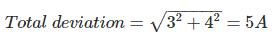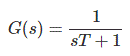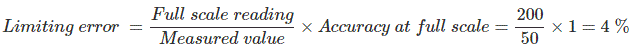# MCQs on Electrical And Electronics Measurements

##### Page 18 of 19. Go to page 1 2 3 4 5 6 7 8 9 10 11 12 13 14 15 16 17 18 19
01․ The total current I = I1 + I2 in a circuit is measured I1 = 150 ± 3A , I2 = 250 ± 4 A, where the limits of error are given as standard deviations. I is measured as
(400 ± 3) A
(400 ± 2.24) A
(400 ±5) A
(400 ± 1) A02․ A first order instrument is characterized by
Static sensitivity and damping coefficient
Time constant only
Static sensitivity and time constant
Static sensitivity, damping coefficient and natural frequency of oscillations

A first order system can be represented asWhere, T is time constant.

03․ The ratio of change in output to the change in the input is called
precision
resolution
sensitivity
repeatability

Sensitivity indicates how promptly an instrument can sense any change in input signal. The ratio of change in output to the change in the input is called sensitivity.

04․ The difference between the indicated value and the true value of a quantity is
Dynamic error
Gross error
Absolute error
Relative error

The difference between the indicated value and the true value or actual value of a quantity is called absolute error.

05․ Which one the following statements is correct? The application of the instrument in wrong manner in the procedure of measurement results in a/an
instrument error
random error
systematic error
gross error

The application of the instrument in wrong manner in the procedure of measurement results in an instrument error. As this error is resulted from instrument itself.

06․ Two capacitance, C1 = 150 ± 2.4µF and C2 = 120 ± 1.5 µF are connected in parallel. What is the limiting error of the resultant capacitance C (in µF) ?
3.9µF
2.4µF
1.5µF
0.9µF

Equivalent capacitance of the given parallel combination is C = C1 + C2. So, limiting error of C is (2.4 + 1.5) = 3.9 µF

07․ Dynamic errors are caused by
instrument not responding fast
human error
environmental error
observational error

Dynamic errors are caused due to sluggish response of instrument.

08․ If two 300V full scale voltmeters V1 and V2 having sensitivities of 100 kOhm/V and 150 kOhm/V are connected in series to measure 500V then
V1 = 200V and V2 = 300V.
V1 = 250V and V2 = 250V.
V1 = 300V and V2 = 200V.
V1 = 0V and V2 = 0V.

The reading of voltmeters will be given as Reading of V1/ Reading of V2 = Sensitivity of V1/ Sensitivity of V2.

09․ A 0 - 200 V voltmeter has a guaranteed accuracy of 1% of full scale reading. The voltage measured by this instrument is 50 V. What is the limiting error?
2%
3%
4%
5%10․ The three resistors R1, R2 and R3 have the following ratings: R1 = 25Ω ± 4%, R2 = 65 Ω ± 4%; R3 =45 Ω ± 4%. If the resistance are connected in series, then limiting error produced in equivalent resistance is
1%
2%
3%
4%

As limiting error of each resistance is ± 4% the limiting error of series combination of all resistance will also be ± 4%

<<<16171819>>>